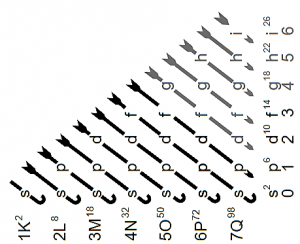Themes

# What is the Moeller diagram?

## Moeller diagram

The  Moeller diagram is a diagram that helps to know the order in which electrons fill the orbitals within an atomic structure . It is composed of 4 columns which represent the orbitals defined by the theory (s, p, d and f, respectively).## What is the Moeller diagram?

With the development of chemistry throughout the nineteenth century , the need for an appropriate theory to explain the details of atomic structure became increasingly latent . In that order, the theory of atomic orbitals was born , which states that electrons remain within a space associated with a given orbit . This implies that the greater the number of electrons in the element, the greater the number of orbitals. In order to express the electronic configuration corresponding to each element, the Moeller diagram was developed, which graphically represents Madelung’s rule.and allows to obtain said configuration quickly and easily. It is also known as the Rain Method .

• What is the Moeller diagram?
• History
• Who invented it
• What is the Moeller diagram for?
• How to do it
• Importance of the Moeller diagram
• Examples

## What is the Moeller diagram?

Determine the order of filling , draw diagonal lines pointing downward, starting on the right and ending on the left. In this way, the beginning of the filling is established in the 1s orbital ; then the electronic filling corresponds to the 2s orbital and so on.

Related Articles

## History

The Moeller diagram follows from Madelung’s Rule , which was developed by the German physicist Erwin Madelung to explain the energy order associated with atomic orbitals . This diagram is also based on the famous Pauli Principle .

## Who invented it

Erwin Madelung   was a German physicist born in Bonn in 1881 and is known as the person in charge of the Madelung Rule , from which the Moeller diagram is derived. His intellectual activity was mainly devoted to the study of atomic physics and quantum mechanics . He was a professor at the Goethe University in Frankfurt and passed away in 1972.

## What is the Moeller diagram for?

This method was developed as a memotechnical rule in such a way that the electronic configuration of an atom can be expressed as a function of the Aufbau principle . This implies that the electrons are integrated in a special order one by one to each available orbital, taking their energy levels as a reference .

In this way, the first orbitals are connected with the lower-energy electrons and the last orbitals are associated with the higher- energy electrons present in the atom.

## How to do it

First, the atomic number Z of the element to be studied must be determined, which is associated with the number of electrons found in a neutral atom . Then, the filling of the electrons in the orbitals is determined by drawing diagonal lines pointing downwards, from left to right.

In this way , the filling is established from the 1s orbital onwards, following the order present in the diagram . It should be noted that, in the case of the “s” orbitals, 2 electrons are housed; in the same way, for the “p” orbitals 6 electrons are housed and 10 electrons for the “d” orbitals, respectively.

An elegant way to rewrite the electron configuration for polyelectronic atoms is this: to rewrite the configuration as that of the noble gas that precedes it plus the next corresponding electrons .

## Importance of the Moeller diagram

The importance of this diagram is that it is an easy and simple way to explain and calculate the electronic configurations of the atom based on Madelung’s Rule . This allowed the massification of chemistry as a study science and was the spearhead for the technological developments that occurred a posteriori.

## Examples

#### Calculation of the electron configuration of Potassium (K) using the Moeller diagram

Initially , the atomic number Z of potassium (K) must be known, which, based on the periodic table, corresponds to Z = 19 . Then, assuming that this value corresponds to the number of electrons that exist in a potassium atom (K) , the indications relative to the drawing of the diagonal lines must be followed to determine the order in which they will be filled in the orbitals. For the case of potassium (K), we obtain: 1s 2 2s 2 2p 6 3s 2 3p 6 4s 1 .

Finally, since potassium (K) is a polyelectronic atom , its electronic configuration can also be rewritten as follows: [Ar] 4s 1 ; In this case, the symbol [Ar] is represented as the electronic configuration associated with the element Argon (Ar) , which is the noble gas that precedes potassium (K) in the periodic table.